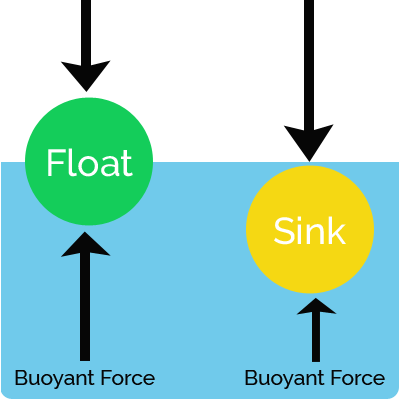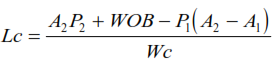# DRILL COLLARS WEIGHT CALCULATIONS | BUOYANCY FACTOR | PRESSURE AREA

## Drill Collars Weight Calculations

###Buoyancy Factor Method

#### Number & Lengths Of Drill Collar Calculations Target & Assumptions

Before going deep in that method, please consider the following notes:

Where, WOB = desired weight on bit, lb  SF= safety factor (1.1-1.15),  BF= buoyancy factor, Wc= drill collar weight in air, lb/ft I= maximum hole angle at BHA, degrees

#### Drill Pipe Buckling

Drill Pipe Buckling is a problem that must be avoided at all times. Heavy Weight Drill Pipe (HWDP) or Drill Pipe Buckling induces stresses in the pipe which will cause premature pipe fatigue and pipe failure.

#### The Drill String Buckling Neutral Point

Lubinski defined The Buckling Neutral Point as the point in the Drill Collar string below which the pipe is buckled (under compression) or will have a tendency to buckle, and above (Also under compression) which no buckling will occur. The buckling neutral point is calculated by the following equation,

Above Equation states that no buckling occurs above the drill collars as long as the weight on the Drilling Bit does not exceed the buoyed weight of the Drill Collar.

#### The Drill String Axial Stress Neutral Point

In some cases it may be necessary to calculate the axial stress in the Drill String or locate the axial stress neutral point. When axial stress must be determined, all forces acting on the Drilling Bottom Hole Assembly BHA must be considered including the hydrostatic forces.
The hydraulic forces are a result of the hydrostatic pressure of the mud and are computed by multiplying the hydrostatic pressure times the respective section area.

### Axial Stresses On Heavy Weight Drill Pipe HWDP  Calculations

To determine the axial stresses in the HWDP above the drill collars, consider the free body diagram

Drilling Bottom Hole Assembly BHA  here consists

The Weight On Drilling Bit WOB acts on the formation, but since for every action there is a reaction equal in magnitude, there will be a reaction force equal to WOB acting upward on the bottom end of the Drill Collars.

Where
• W1 = LH WH
• W2 = LC WC
• F1   = P1 (A2-A1)
• F2   = P2 A2

Where WH  = weight in air of HWDP, lb/ft
WC  = weight in air of drill collars, lb/ft
LH    = length of HWDP, ft
LC    = length of drill collars, ft
P1    = hydrostatic pressure at top of drill collars, psi
P2    = hydrostatic pressure at bottom of drill collars, psi
A1   = steel cross sectional area for HWDP, in2
A2   = steel cross section area for drill collars, in2
FT   = tensile force, lb
It should be noted that FT is assumed to be a tensile force. If the magnitude of FT is negative then it is a compressive force.
To determine the axial stresses in the drill collars consider the free body diagram. Note that in this case there is no hydrostatic force acting on the top of the drill collars because there is no change in diameter (no shoulder area). Adding the forces gives,

Drill Collar Calculation Pressure Area Method Conclusion

### Pressure Area Method For Drill Collar Calculation

The Pressure-Area Method takes into account all the forces acting on the Drilling Bottom Hole Assembly BHA including the hydraulic forces. Consider the free body diagram in above Figure. A force balance yields,The length of Drill Collar required to provide Weight On Drilling Bit WOB is,## Drill Collars Weight Calculations | Buoyancy Factor Method Vs Pressure Area Method

### Comparison between the Buoyancy Factor Method and the Pressure Area Method for the Drill Collar weight calculations.

• The buckling neutral point is the same in both cases.
• Either of the two methods can be used to calculate the length of the Drill Collar

### However, the Pressure Area Method has the following disadvantages:

• Requires more Drill Collars to keep the Heavy Weight Drill Pipe HWDP or Drill Pipes in tension. This serves no useful purpose because whether the pipe above the buckling neutral point is in tension or compression is irrelevant to fatigue damage, if the pipe is not buckled.
• The need to procure, transport, maintain, inspect and handle the extra Drill Collars increase the cost of the drilling operation